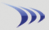Subscription & data-feed pricing Class schedule

Margin rates Stock & option commissions

Attention: Discussion forums are read-only for extended maintenance until further notice.

 Boolean Rate this Topic:Previous Topic · Next Topic Watch this topic · Print this topic ·
Quiktdr
 Posted : Friday, January 18, 2019 1:39:27 PMPlatinum Customer

Joined: 10/7/2004
Posts: 794

I enter this as a Boolean but it keeps telling me it has to be a Boolean

{ATR} (((((H - L) * ((H - L) >= ABS((H - C1))))) + ((ABS((C1 - H)) * ( ABS((H - C1)) > (H - L))))) * (((((L - H) * ((H - L) >= ABS((H - C1))))) + (( - ABS((C1 - H)) * ( ABS((H - C1)) > (H - L))))) >= ABS((C1 - L)))) + (( - ABS(C1 - L)) * (ABS(C1 - L) > ((((L - H) * ((H - L) > ABS((H - C1))))) + (( - ABS((C1 - H)) * ( ABS((H - C1)) > (H - L)))))))

StockGuy
 Posted : Friday, January 18, 2019 1:41:32 PMJoined: 9/30/2004
Posts: 9,187

You need to pair the booleans with ANDs.

A > B and B > C

A > B > C is not valid.

Quiktdr
 Posted : Friday, January 18, 2019 1:54:58 PMPlatinum Customer

Joined: 10/7/2004
Posts: 794

Thisis the formula I received on forum for ATR. Is it incorrect?

StockGuy
 Posted : Friday, January 18, 2019 1:57:53 PM